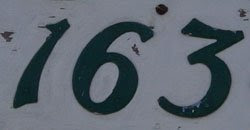## Tuesday, May 12, 2009

### 163

163 is a prime number.

163 is the smallest three-digit number whose square (26569) and cube (4330747) use different digits.

163 is conjectured to be the largest prime that can be represented uniquely as the sum of three squares (if squares are allowed to be zero): 163 = 12 + 92 + 92.

163 has a unique representation as a sum of three squares: 163 = 12 + 92 + 92.163 is the smallest score impossible to make using up to but not more than three darts.

Source:
Prime Curios!